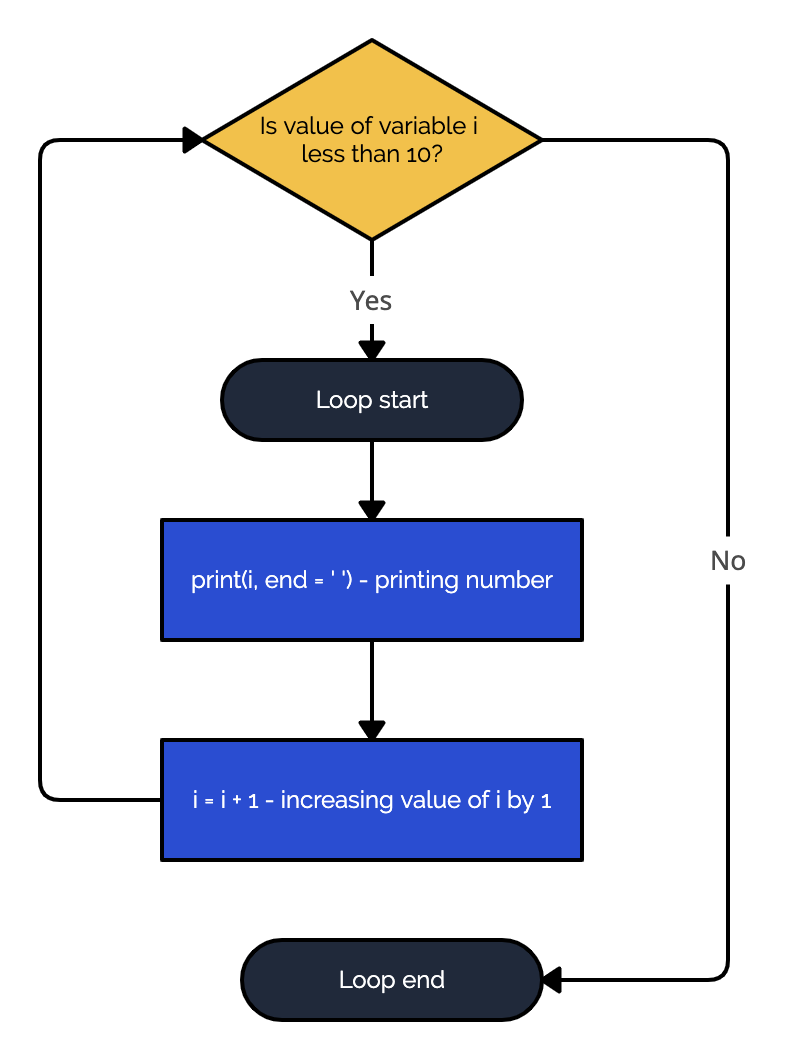Course Content

Introduction to Pythonwhile Loop

Often, you need your code to iterate while a particular condition holds. For example, in real life, we don't quit the metro train till we reach our station, i.e., if we need "Station B," we will skip "Station A," "Station C," and so on until we reach "Station B." In Python, you can write something like this using a `while` loop:For example, we can print all the numbers to 10.The algorithm of the loop is shown above. You might notice that we use `i = i + 1` inside the loop. If we didn't specify that, our loop would be infinite, as every time it checks the condition, it gets `1 < 10`, which is True. When using this type of loop, be careful that your code execution doesn't become infinite.

Section 5.

Chapter 1